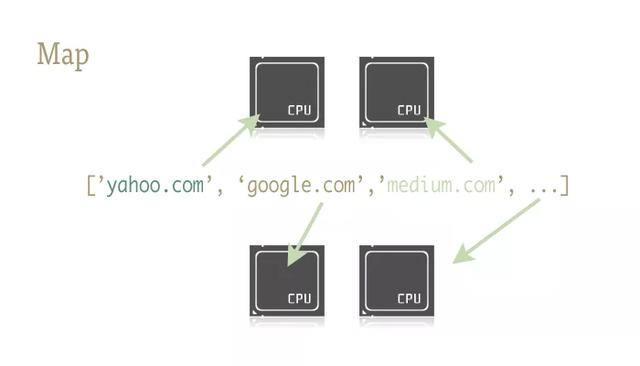# 怎样用一行 Python 代码实现并行

Python 在程序并行化方面多少有些声名狼藉。撇开技术上的问题，例如线程的实现和 GIL，我觉得错误的教学指导才是主要问题。常见的经典 Python 多线程、多进程教程多显得偏"重"。而且往往隔靴搔痒，没有深入探讨日常工作中最有用的内容。

``````import os
import PIL

from multiprocessing import Pool
from PIL import Image

SIZE = (
75,
75)
SAVE_DIRECTORY =  thumbs

def get_image_paths(folder):

return (
os.
path.join(folder, f)

for f
in
os.listdir(folder)

if  jpeg
in f)

def create_thumbnail(filename):
im = Image.
open(filename)
im.thumbnail(SIZE, Image.ANTIALIAS)
base, fname =
os.
path.split(filename)
save_path =
os.
path.join(base, SAVE_DIRECTORY, fname)
im.save(save_path)

if __name__ ==  __main__ :
folder =
os.
path.abspath(

11_18_2013_R000_IQM_Big_Sur_Mon__e10d1958e7b766c3e840 )

os.mkdir(
os.
path.join(folder, SAVE_DIRECTORY))

images = get_image_paths(folder)

pool = Pool()
pool.map(creat_thumbnail, images)
pool.
close()
pool.join()``````

but...问题在于…

worker 越多，问题越多

``````#Example2.py

A more realistic thread pool example

import time
import Queue
import urllib2

def __init__(
self, queue):
self)

self._queue = queue

def run(
self):

while True:
content =
self._queue.get()

if isinstance(content,
str) and content ==  quit :

break
response = urllib2.urlopen(content)
print  Bye byes!

def Producer():
urls = [
http:
// ,

,

# etc..

]

queue = Queue.Queue()

start_time = time.time()

# Add the urls to process

for url in urls:

queue.put(url)

queue.put( quit )

worker.join()

print  Done! Time taken: {} .format(time.time() - start_time)

def build_worker_pool(queue, size):

workers = []

for _ in range(size):

worker = Consumer(queue)

worker.start()

workers.append(worker)

return workers

if __name__ ==  __main__ :

Producer()``````

map 这一小巧精致的函数是简捷实现 Python 程序并行化的关键。map 源于 Lisp 这类函数式编程语言。它可以通过一个序列实现两个函数之间的映射。

``````
urls = [  ,   ]
results = map(urllib2.urlopen, urls)``````

``````
results = []
for url in urls:
results.append(urllib2.urlopen(url))``````

map 函数一手包办了序列操作、参数传递和结果保存等一系列的操作。dummy 是 multiprocessing 模块的完整克隆，唯一的不同在于 multiprocessing 作用于进程，而 dummy 模块作用于线程（因此也包括了 Python 所有常见的多线程限制）。

``````
from multiprocessing
import Pool
from multiprocessing.dummy
import Pool

`pool = ThreadPool()`

Pool 对象有一些参数，这里我所需要关注的只是它的第一个参数：processes. 这一参数用于设定线程池中的线程数。其默认值为当前机器 CPU 的核数。

`pool = ThreadPool(4) # Sets the pool size to 4`

``````import urllib2
from multiprocessing.dummy import Pool as ThreadPool

urls = [

http:/
/ ,

http:/
/ ,

http:/
/
/a/python
/2003/
04/
17/metaclasses.html ,

http:/
//doc
/ ,

http:/
/ ,

http:/
//getit
/ ,

http:/
//community
/ ,

https:/
/wiki.python.org/moin
/ ,

http:/
/planet.python.org/ ,

https:/
/wiki.python.org/moin
/LocalUserGroups ,

http:/
//psf
/ ,

http:/
/docs.python.org/devguide
/ ,

http:/
//community
/awards/

# etc..

]

# Make the Pool of workers

# Open the urls in their own threads

# and return the results

results = pool.map(urllib2.urlopen, urls)

#close the pool and wait for the work to finish

pool.close()

pool.join()``````

``````# results = []
#
for url in urls:
#   result = urllib2.urlopen(url)
#   results.
append(result)

# # ------- VERSUS ------- #

# # -------
4 Pool ------- #
4)
# results = pool.
map(urllib2.urlopen, urls)

# # -------
8 Pool ------- #

8)
# results = pool.
map(urllib2.urlopen, urls)

# # -------
13 Pool ------- #

13)
# results = pool.
map(urllib2.urlopen, urls)``````

``````#
Single
.4
Seconds
#               4
Pool:   3
.1
Seconds
#               8
Pool:   1
.4
Seconds
#              13
Pool:   1
.3
Seconds``````

``````import os
import PIL

from multiprocessing import Pool
from PIL import Image

SIZE = (
75,
75)
SAVE_DIRECTORY =  thumbs

def get_image_paths(folder):

return (
os.
path.join(folder, f)

for f
in
os.listdir(folder)

if  jpeg
in f)

def create_thumbnail(filename):
im = Image.
open(filename)
im.thumbnail(SIZE, Image.ANTIALIAS)
base, fname =
os.
path.split(filename)
save_path =
os.
path.join(base, SAVE_DIRECTORY, fname)
im.save(save_path)

if __name__ ==  __main__ :
folder =
os.
path.abspath(

11_18_2013_R000_IQM_Big_Sur_Mon__e10d1958e7b766c3e840 )

os.mkdir(
os.
path.join(folder, SAVE_DIRECTORY))

images = get_image_paths(folder)

for image
in images:
create_thumbnail(Image)``````

``````import os
import PIL

from multiprocessing import Pool
from PIL import Image

SIZE = (
75,
75)
SAVE_DIRECTORY =  thumbs

def get_image_paths(folder):

return (
os.
path.join(folder, f)

for f
in
os.listdir(folder)

if  jpeg
in f)

def create_thumbnail(filename):
im = Image.
open(filename)
im.thumbnail(SIZE, Image.ANTIALIAS)
base, fname =
os.
path.split(filename)
save_path =
os.
path.join(base, SAVE_DIRECTORY, fname)
im.save(save_path)

if __name__ ==  __main__ :
folder =
os.
path.abspath(

11_18_2013_R000_IQM_Big_Sur_Mon__e10d1958e7b766c3e840 )

os.mkdir(
os.
path.join(folder, SAVE_DIRECTORY))

images = get_image_paths(folder)

pool = Pool()
pool.map(creat_thumbnail, images)
pool.
close()
pool.join()``````

5.6 秒！

• 博文量
995
• 访问量
622942